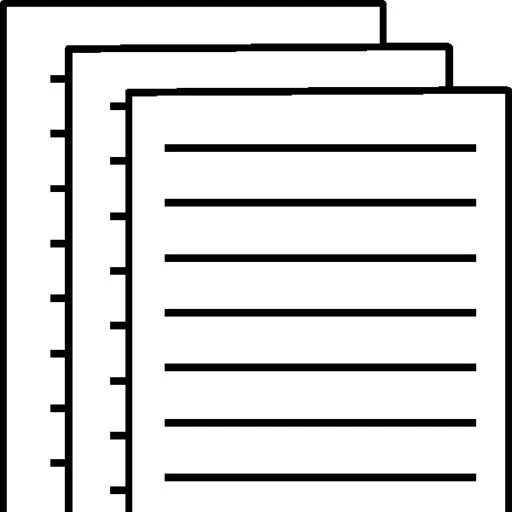## Trigonometry Assignment 1

4 Oct No Comments

Assignment #1

Answer each of the following questions. You must provide detailed work illustrating how you arrived at your solution.

Submit your answers to the drop box.

Your work may be attached as a Word Document or a PDF File.

1) An open box with a square base (see figure) is to be constructed from 108 square inches of material. The height of the box is 3 inches. What are the dimensions of the box?Surface area = 108 square inches

X*X + 4*a*x = 108

X2 +12x=108

X2 +12x-108=0

Solve the quadratic equation

D=b2-4ac=122 – 4 *1* -108=576

X= (-b±√D)/(2*a)

X=(-12±√576)/(2*1)

X= 6 or -18.

Use the positive value

Dimensions = 6*6*3

2) An electric hoist is being used to lift a beam. The diameter of the drum on the hoist is 13 inches, and the beam must be raised 3 feet. Find the number of degrees through which the drum must rotate. Round your answer to nearest whole number. We are given a = 13 and b = 3.

Circumference = 3

Circumference = (θ/360)* ∏*D

Θ=(360 * Circumference)/( ∏*D)

Θ=(360*3)/(13*22/7)

= 1080/40.857

= 26 degrees

3) The hypotenuse of an isosceles right triangle is 4 centimeters long. How long are its sides? Round your answers to two decimal places.

Isosceles – two equal sides

Assume opposite an adjacent are equal. The angles between hypotenuse and the two are equal.

180-90=90/2=45degrees each.

Cos 45 = adj/4

Adj = 4 * 0.7071

=2.83cm

4) A sprinkler on a golf green is set to spray water over a distance of 12 meters and to rotate through an angle of 140°. Find the area of the region. Round your answer to two decimal places.

Sector sprinkled= 140/360

∏ = 22/7

Area=θ/360* ∏ * R2

= 140/360*22/7*12*12

= 176.00M2

Grading Criteria Assignments Maximum Points 40 20 30 10 100

To view and download a complete answer, scroll down to the bottom to pay